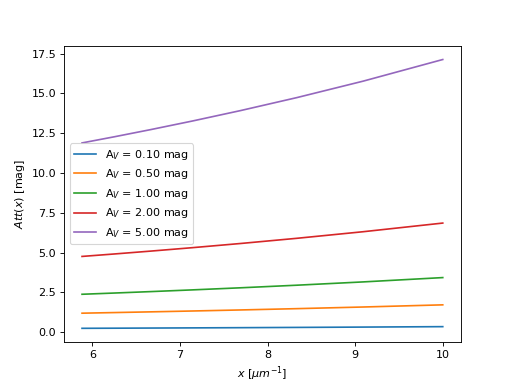# L02¶

class dust_attenuation.averages.L02(*args, meta=None, name=None, **kwargs)[source]

Attenuation curve of Leitherer et al. (2002). Narrow validity range: 0.097 to 0.18 microns

Parameters
Av: float

attenuation in V band

Raises
InputParameterError

Input Av values outside of defined range

Notes

From Leitherer (2002, ApJS, Volume 140, Issue 2, pp. 303-329), eq. 14

Example:

import numpy as np
import matplotlib.pyplot as plt
import astropy.units as u

from dust_attenuation.averages import L02

fig, ax = plt.subplots()

# generate the curves and plot them
x = np.arange(0.1,0.18,0.01)*u.micron

Avs = [0.1,0.5,1.0,2.0,5.0]
for cur_Av in Avs:
att_model = L02(Av=cur_Av)
ax.plot(1/x,att_model(x),label=r'A$_V$ = %.2f mag' % (cur_Av))

ax.set_xlabel(r'$x$ [$\mu m^{-1}$]')
ax.set_ylabel(r'$Att(x)$ [mag]')

ax.legend(loc='best')
plt.show()Attributes Summary

Methods Summary

 evaluate(x, Av) Returns the attenuation curve, A(λ), following the recipe of Leitherer et al. Compute the starburst reddening curve of Leitherer et al. (2002)

Attributes Documentation

Rv = 4.05
x_range = [0.097, 0.18]

Methods Documentation

evaluate(x, Av)[source]

Returns the attenuation curve, A(λ), following the recipe of Leitherer et al. (2002), assuming Rv=4.05

Parameters
in_x: float

expects either x in units of wavelengths or frequency or assumes wavelengths in [micron]

internally microns are used

Returns
att: np array (float)

Att(x) attenuation curve [mag]

Raises
ValueError

Input x values outside of defined range

k_lambda(x)[source]
Compute the starburst reddening curve of Leitherer et al. (2002)

k’(λ)=A(λ)/E(B-V)

Parameters
in_x: float

expects either x in units of wavelengths or frequency or assumes wavelengths in [micron]

internally microns are used

Returns
k_lambda: np array (float)

k_lambda(x) reddening curve

Raises
ValueError

Input x values outside of defined range